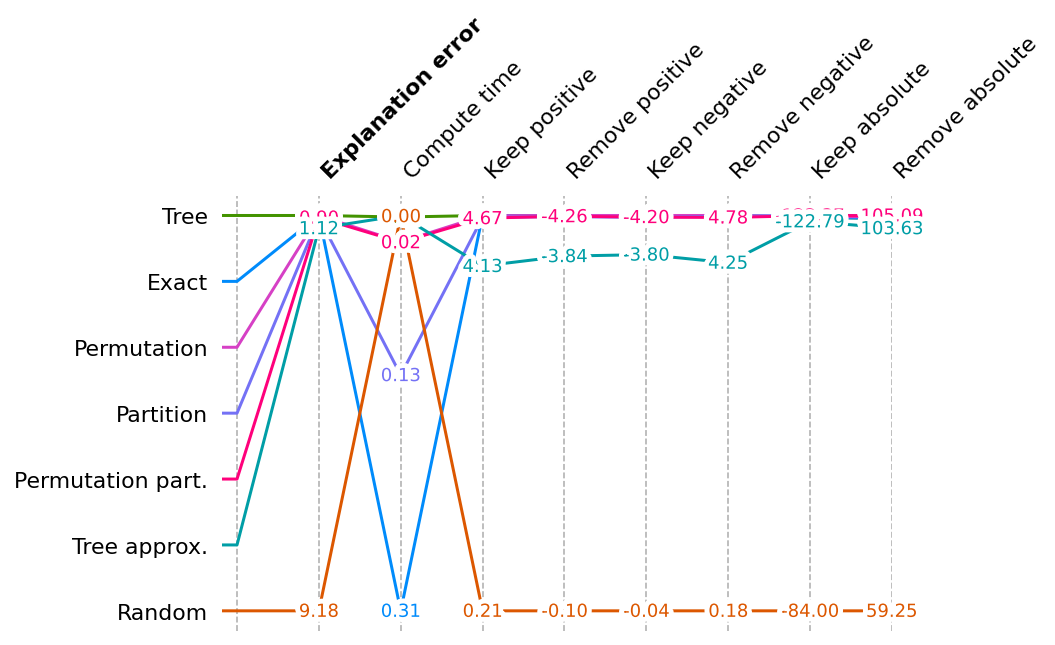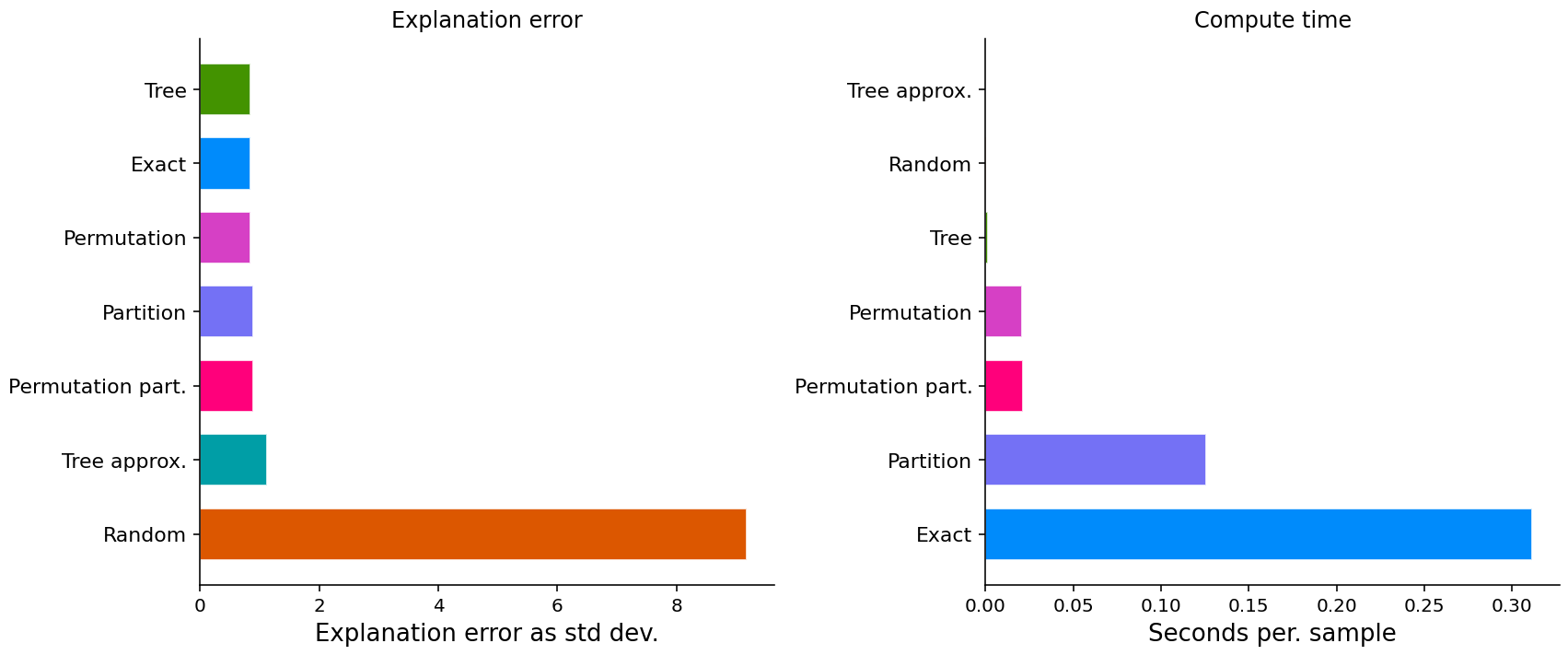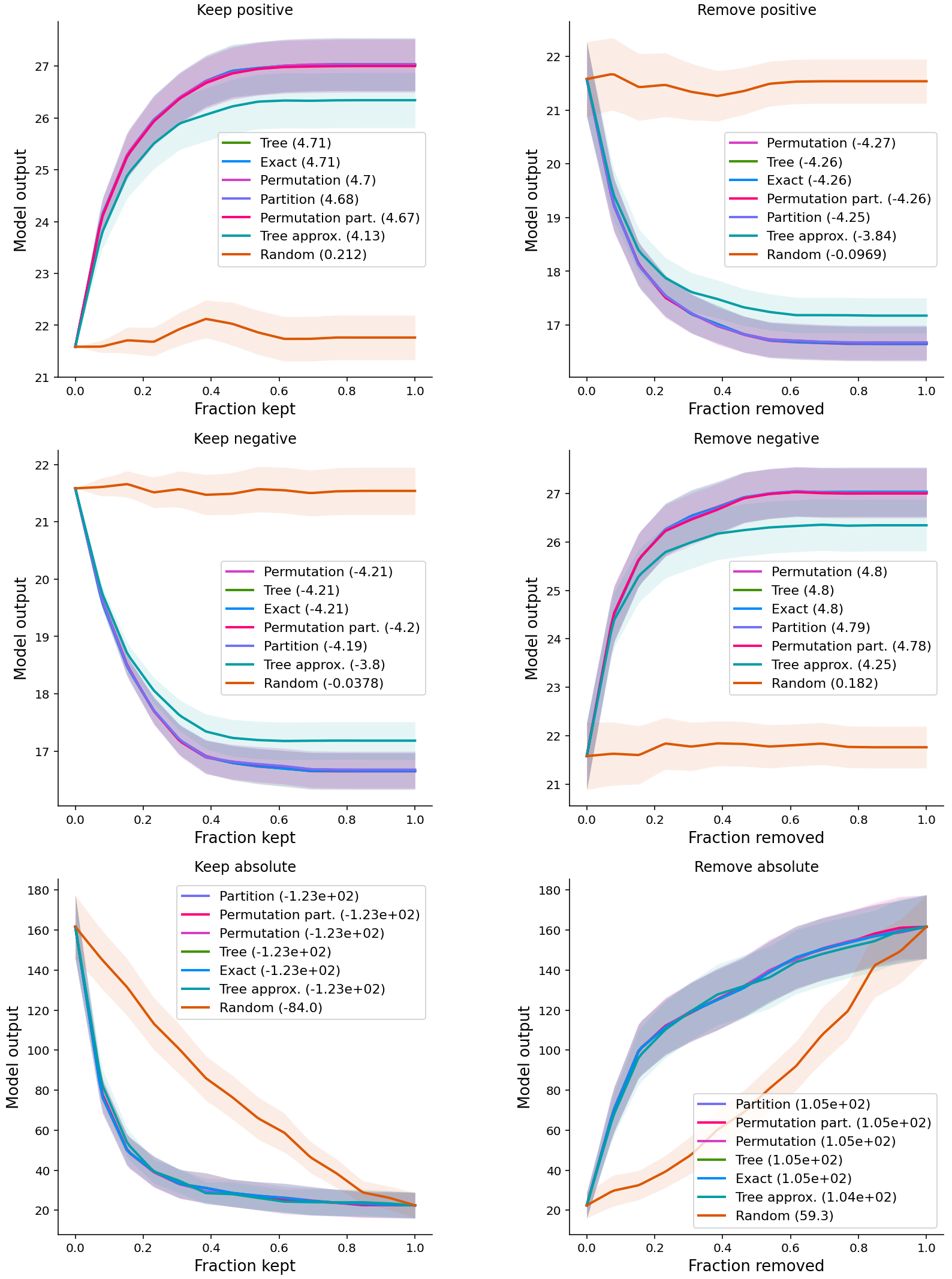# SHAP源码之Benchmark示例

Contents

## Benchmark XGBoost explanations

These benchmark notebooks compare different types of explainers across a variety of metrics. They are all generated from Jupyter notebooks available on GitHub.

• Model: XGBoost
• Dataset: Boston Housing (Tabular)

## Build Explainers

``````# use an independent masker

# build the explainers
explainers = [
]
``````

``````# shap/maskers/__init__.py
from ._tabular import Independent, Partition, Impute
from ._image import Image
from ._text import Text
from ._fixed import Fixed
from ._composite import Composite
from ._fixed_composite import FixedComposite
from ._output_composite import OutputComposite
``````

The two types of masker used during building the explainers:

• masker: `Independent` masks out tabular features by integrating over the given background dataset.
• pmasker: `Partition` Unlike Independent, Partition respects a hierarchial structure of the data.
• param `clustering`string (distance metric to use for creating the clustering of the features) or numpy.ndarray (the clustering of the features).

The following two types of masker is used during benchmarking:

• cmasker: `Composite` merges several maskers for different inputs together into a single composite masker.
• `Fixed` leaves the input unchanged during masking, and is used for things like scoring labels.

### shap.explainers

``````# shap/explainers/__init__.py
from ._permutation import Permutation
from ._partition import Partition
from ._tree import Tree
from ._gpu_tree import GPUTree
from ._exact import Exact
from ._linear import Linear
from ._sampling import Sampling
from ._deep import Deep
``````
``````# shap/__init__.py

# explainers
from .explainers._explainer import Explainer
from .explainers._kernel import Kernel as KernelExplainer
from .explainers._sampling import Sampling as SamplingExplainer
from .explainers._tree import Tree as TreeExplainer
from .explainers._gpu_tree import GPUTree as GPUTreeExplainer
from .explainers._deep import Deep as DeepExplainer
from .explainers._linear import Linear as LinearExplainer
from .explainers._partition import Partition as PartitionExplainer
from .explainers._permutation import Permutation as PermutationExplainer
from .explainers import other # Coefficent, Random, LimeTabular, Maple, TreeMaple, TreeGain
``````

The `explainers` list is made up of 6 explainers of 4 types (5 classes):

• model-agnostic
• “Permutation”, “Permutation part.”: `Permutation` (masker = masker/pmasker) approximates the Shapley values by iterating through permutations of the inputs.
• “Partition”: `Partition` has two particularly nice properties
• model-agnostic but when using a balanced partition tree only has quadradic exact runtime (in term of the number of input features). This is in contrast to the exponential exact runtime of KernalExplainer or SamplingExplainer.
• always assigns to groups of correlated features the credit that set of features would have had if treated as a group
• tree
• Tree”, “Tree approx.”: `Tree` (approximate = False/True)
• shapley
• “Exact”: `Exact` computes SHAP values via an optimized exact enumeration.
• baseline
• “Random”: `Random` simply returns random (normally distributed) feature attributions.

## Run Benchmarks

``````# explain with all the explainers
attributions = [(name, exp(X_eval)) for name, exp in explainers]

results = {} # metric name: shap.benchmark._result.BenchmarkResult
``````

`results` is a dict that stores metric name and the corresponding list of `shap.benchmark._result.BenchmarkResult` for the list of explainers.

### shap.benchmark

``````# shap/benchmark/__init__.py
from ._result import BenchmarkResult
from ._compute import ComputeTime
from ._explanation_error import ExplanationError
``````

The following three metrics are used in the experiments:

• `ExplanationError`: A measure of the explanation error relative to a model’s actual output.
• For explanations (like Shapley values) that explain the difference between one alternative and another (for example a current sample and typical background feature values) there is possible explanation error for every pattern of mixing foreground and background (i.e., every possible masking pattern).
• compute the standard deviation over these explanation errors where masking patterns are drawn from prefixes of random feature permutations.
• __call__: explanation, name, step_fraction=0.01, indices=[], silent=False
``````smasker = shap.benchmark.ExplanationError(
) # __init__
``````
``````ct = shap.benchmark.ComputeTime()
results["compute time"] = [ct(v, name=n) for n,v in attributions]
``````
• `SequentialMasker`: auc score after feature perturbations
• __call__: explanation, name, **kwargs
``````for mask_type, ordering in [("keep", "positive"), ("remove", "positive"), ("keep", "negative"), ("remove", "negative")]:
)

for mask_type, ordering in [("keep", "absolute"), ("remove", "absolute")]:
)
``````

## Visualize Results

### shap.plots.benchmark

#### Show scores across all metrics for all explainers

This multi-metric benchmark plot sorts the method by the first method, and rescales the scores to be relative for each metric, so that the best score appears at the top and the worse score at the bottom.

``````# accept a list of BenchmarkResult
shap.plots.benchmark(sum(results.values(), []))
# plot without Random
shap.plots.benchmark(filter(lambda x: x.method != 'Random', sum(results.values(), [])))
``````#### Show detail plots of each metric type

If we plot scores for one metric at a time then we can see a much more detailed comparison of the methods.

``````import matplotlib.pyplot as plt
num_plot_rows = len(results) // 2 + len(results) % 2
fig, ax = plt.subplots(num_plot_rows, 2, figsize=(12, 5 * num_plot_rows))

for i, k in enumerate(results):
plt.subplot(num_plot_rows, 2, i+1)
shap.plots.benchmark(results[k], show=False)
if i % 2 == 0:
ax[-1, -1].axis('off')
plt.tight_layout()
plt.show()
``````
• Some methods just have a score (explanation error and compute time).• Other methods have entire performance curves, and the score is the area under (or over) these curves.• Postive: important features; Negative: unimportant features
• For the first two lines of curves, the output is the original output of XGBoost
• For the last line of curve, the output is `(y - model.predict(X))**2`
Updated on